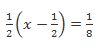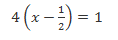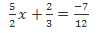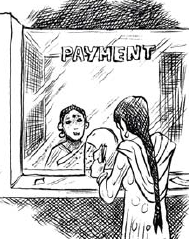# NCERT Solutions for Linear equations Class 8 maths Chapter 2 Exercise 2.2

In this page we have NCERT Solutions for Linear equations Class 8 maths Chapter 2 Exercise 2.2.This exercise has questions about some applications on simple linear equation.Here you need to form the equation from question and then solve it. Hope you like them and do not forget to like , social share and comment at the end of the page.

## NCERT Solutions for Linear equations Class 8 maths Chapter 2 Exercise 2.2

Question 1:
If you subtract ½ from a number and multiply the result by 1/2, you get 1/8. What is the number?
Let x be the number then as per the questionThe LCM of denominator is 8, so multiplying by 8 on both sides4x-2=1
Transposing 2 to R.H.S, we obtain
4x=3
Dividing 4 on both the sides
x=3/4

Question 2:
The perimeter of a rectangular swimming pool is 154 m. Its length is 2 m more than twice its breadth. What are the length and the breadth of the pool?
Let the breadth be x m.
Then as per question the length will be (2x+ 2) m.
Perimeter of swimming pool = 2(l + b) = 154 m
$2(2x+ 2 +x) = 154$
$2(3x+ 2) = 154$
Dividing both sides by 2
$3x+ 2 = 77$
Transposing 2 to R.H.S, we obtain
$3x= 77 - 2$
3x= 75
Dividing 3 on both the sides
x= 25
So
Breath is 25 m
Length =2x+ 2 = 2 × 25 + 2 = 52m
Hence, the breadth and length of the pool are 25 m and 52 m respectively.

Question 3:
The base of an isosceles triangle is 4/3 cm. The perimeter of the triangle is $4 \frac {2}{15}$ cm. What is the length of either of the remaining equal sides?

Let the length of equal sides be x cm.
Perimeter =x+ x + Base
Substituting the values, we getThe LCM of the denominator is 15, so multiplying both the sides by 15
$30x+ 20=62$
Transposing 20 to R.H.S, we obtain
30x=42
Dividing by 30 on both the sides
x=42/30=7/5
Therefore, the length of equal sides is 7/5 cm.

Question 4:
Sum of two numbers is 95. If one exceeds the other by 15, find the numbers.
Let one number be x. Therefore,the other number will be x+ 15.
According to the question,
$x + x+ 15 = 95$
$2x+ 15 = 95$
Transposing 15 to R.H.S, we obtain
$2x= 95 - 15$
2x= 80
Dividing both sides by 2, we obtain
x= 40
So the other number will be
$x+ 15 = 40 + 15 = 55$
Hence, the numbers are 40 and 55.

Question 5:
Two numbers are in the ratio 5:3. If they differ by 18, what are the numbers?
In this type of question, the strategy is to choose common ratio and solve the equation to find the exact numbers
Let the common ratio between these numbers be x. Therefore, the numbers will be 5x and 3x respectively.
Difference between these numbers = 18
$5x - 3x= 18$
2x= 18
Dividing both sides by 2,
x= 9
First number = 5x= 5 × 9 = 45
Second number = 3x= 3 × 9 = 27

Question 6:
Three consecutive integers add up to 51. What are these integers?
Let three consecutive integers be x,x+ 1, andx+ 2.
Then as per question
Sum of these numbers= x+ x+ 1 +x+ 2 = 51
$3x+ 3 = 51$
Transposing 3 to R.H.S, we obtain
$3x= 51 - 3$
$3x= 48$
Dividing both sides by 3
x= 16, so other numbers will be
$x+ 1 = 17$
$x+ 2 = 18$
Hence, the consecutive integers are 16, 17, and 18.

Question 7:
The sum of three consecutive multiples of 8 is 888. Find the multiples.
Let the three consecutive multiples of 8 be 8x, 8(x+ 1), 8(x+ 2).
As per questions,
Sum of these numbers=8x+ 8(x+ 1) + 8(x+ 2) = 888
$8(x+x+ 1 +x+ 2) = 888$
$8(3x+ 3) = 888$
Dividing both sides by 8
$3x+ 3 = 111$
Transposing 3 to R.H.S, we obtain
$3x= 111 - 3$
3x= 108
Dividing both sides by 3, we obtain
x= 36, so the three multiples will be
First multiple =8x =8 × 36 = 288
Second multiple =8(x+ 1) = 8 × (36 + 1) = 8 × 37 = 296
Third multiple =8(x+ 2) = 8 × (36 + 2) = 8 × 38 = 304
Hence, the required numbers are 288, 296, and 304.

Question 8 :
Three consecutive integers are such that when they are taken in increasing order and multiplied by 2, 3 and 4 respectively, they add up to 74. Find these numbers.

Let three consecutive integers be x,x+ 1,x+ 2.
According to the question,
$2x+ 3(x+ 1) + 4(x+ 2) = 74$
$2x+ 3x+ 3 + 4x+ 8 = 74$
$9x+ 11 = 74$
Transposing 11 to R.H.S, we obtain
$9x= 74 - 11$
$9x= 63$
Dividing both sides by 9, we obtain
x= 7, so the integers are
x+ 1 = 7 + 1 = 8
x+ 2 = 7 + 2 = 9
Hence, the numbers are 7, 8, and 9.

Question 9 :
The ages of Rahul and Haroon are in the ratio 5:7. Four years later the sum of their ages will be 56 years. What are their present ages?
In this type of question, the strategy is to choose common ratio and solve the equation to find the exact numbers
Let common ratio between Rahul's age and Haroon's age be y.
Then
Age of Rahul =5y years
Age of haroon = 7y years
So After 4 years, the age of Rahul and Haroon will be (5y+ 4) years and (7y+ 4) years respectively.
According to the given question, after 4 years, the sum of the ages of Rahul and Haroon is 56 years.
$(5y+ 4) + (7y+ 4) = 56$
$12y+ 8 = 56$
Transposing 8 to R.H.S, we obtain
$12y= 56 - 8$
12y= 48
Dividing both sides by 12
y= 4,so the ages will be
Rahul’s age = 5y years = (5 × 4) years = 20 years
Haroon’s age = 7y years = (7 × 4) years = 28 years

Question 10 :
The number of boys and girls in a class are in the ratio 7:5. The number of boys is 8 more than the number of girls. What is the total class strength?
Let the common ratio between the number of boys and numbers of girls be y
Number of boys = 7y
Number of girls = 5y
As per the question,
Number of boys = Number of girls + 8
$7y= 5y+ 8$
Transposing 5y to L.H.S, we obtain
$7y - 5y= 8$
2y= 8
Dividing both sides by 2
y= 4
So the strength of boys and girls are
Number of boys = 7y= 7 × 4 = 28
Number of girls = 5y= 5 × 4 = 20
Hence, total class strength = 28 + 20 = 48 students

Question 11 :
Baichung's father is 26 years younger than Baichung's grandfather and 29 years older than Baichung. The sum of the ages of all the three is 135 years. What is the age of each one of them?

Let us assume Baichung’s father’s age be x years.
Therefore, Baichung’s age and Baichung’s grandfather’s age will be (x- 29) years and (x+ 26) years respectively.
As per the question,
The sum of the ages of these 3 people is 135 years.
So
$x +( x - 29) +(x+ 26) = 135$
$3x- 3 = 135$
Transposing 3 to R.H.S, we obtain
$3x= 135 + 3$
3x= 138
Dividing both sides by 3
x= 46, then the ages of three people will be
Baichung’s father’s age =x years = 46 years
Baichung’s age = (x- 29) years = (46 - 29) years = 17 years
Baichung’s grandfather’s age = (x+ 26) years = (46 + 26) years = 72 years

Question 12 :
Fifteen years from now Ravi’s age will be four times his present age. What is Ravi’s present age?
Let Ravi’s present age be x years.
Fifteen years later, Ravi’s age = 4 × His present age
$x+ 15 = 4x$
Transposing x to R.H.S, we obtain
$15 = 4x-x$
15 = 3x
Dividing both sides by 3, we obtain
5 =x
Hence, Ravi’s present age = 5 years

Question 13 :
A rational number is such that when you multiply it by 5/2 and add 2/3 to the product, you get -7/12. What is the number?

Let x be the rational number ,thenThe LCM of 2,3 and 12 is 12,so multiplying by 12
$30x+8=-7$
Transposing 8 to R.H.S, we obtain
$30x=-15$
Dividing by 30 on both the sides
x=-1/2

Question 14
Lakshmi is a cashier in a bank. She has currency notes of denominations Rs 100, Rs 50 and Rs 10, respectively. The ratio of the number of these notes is 2:3:5. The total cash with Lakshmi is Rs 4, 00,000. How many notes of each denomination does she have?Let the common ratio between the numbers of notes of different denominations be y. Therefore, numbers of Rs 100 notes, Rs 50 notes, and Rs 10 notes will be 2y, 3y, and 5y respectively.
Amount of Rs 100 notes = Rs (100 × 2y) = Rs 200y
Amount of Rs 50 notes = Rs (50 × 3y)= Rs 150y
Amount of Rs 10 notes = Rs (10 × 5y) = Rs 50y
As per question the total amount is Rs 400000.
$200x+ 150x+ 50x= 400000$
400x= 400000
Dividing both sides by 400, we obtain
x= 1000,so number of notes of each denominations
Number of Rs 100 notes = 2x= 2 × 1000 = 2000
Number of Rs 50 notes = 3x= 3 × 1000 = 3000
Number of Rs 10 notes = 5x= 5 × 1000 = 5000

Question 15:
I have a total of Rs 300 in coins of denomination Re 1, Rs 2 and Rs 5. The number of Rs 2 coins is 3 times the number of Rs 5 coins. The total number of coins is 160. How many coins of each denomination are with me?

Let the number of Rs 5 coins be x.
Then Number of Rs 2 coins = 3x
Now it is given that that total number of coins is 160,so
Number of Re 1 coins = 160 - (Number of coins of Rs 5 and of Rs 2)
= 160 - (3x+x) = 160 - 4x
Amount of Re 1 coins = Rs [1 × (160 - 4x)] = Rs (160 - 4x)
Amount of Rs 2 coins = Rs (2 × 3x)= Rs 6x
Amount of Rs 5 coins = Rs (5 ×x) = Rs 5x
As per question total amount is Rs 300.
$160 - 4x+ 6x+ 5x= 300$
$160 + 7x= 300$
Transposing 160 to R.H.S, we obtain
7x= 300 - 160
7x= 140
Dividing both sides by 7, we obtain
x= 20, so the number of coins of each denominations will be
Number of Re 1 coins = 160 - 4x= 160 - 4 × 20 = 160 - 80 = 80
Number of Rs 2 coins = 3x= 3 × 20 = 60
Number of Rs 5 coins =x= 20

Question 16 :
The organizers of an essay competition decide that a winner in the competition gets a prize of Rs 100 and a participant who does not win gets a prize of Rs 25. The total prize money distributed is Rs 3000. Find the number of winners, if the total number of participants is 63.
Let the number of winners be x.
Therefore, the number of participants who did not win will be 63 -x.
Amount given to the winners = Rs (100 ×x) = Rs 100x
Amount given to the participants who did not win = Rs [25(63 -x)]
= Rs (1575 - 25x)
As per the question,
$100x+ 1575 - 25x= 3000$
Transposing 1575 to R.H.S, we obtain
$75x= 3000 - 1575$
$75x= 1425$
Dividing both sides by 75, we obtain
x= 19
Hence, number of winners = 19

## Summary

1. NCERT Solutions for Linear equations Class 8 maths Chapter 2 Exercise 2.2 has been prepared by Expert with utmost care. If you find any mistake.Please do provide feedback on mail. You can download the solutions as PDF in the below Link also
2. This chapter 2 has total 6 Exercise 2.1,2.2,2.3,2.4,2.5.2.6. This is the Second exercise in the chapter.You can explore previous exercise of this chapter by clicking the link below